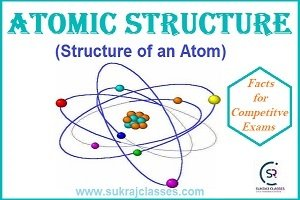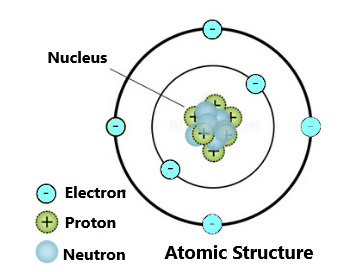# Atomic Structure – Structure of an Atom

Chemistry topic- “Atomic Structure – Structure of an Atom” is important for all competitive exams like CET- Common eligibility Test,  SSC CGL, SSC CHSL, RRB NTPC, UPSC and other state civil services exams. In these exams, almost 4-5 questions are coming from Chemistry. Let’s start the topic: Atomic Structure – Structure of an Atom.

## Structure of an Atom

Atomic Structure – Structure of an Atom

Atomic Structure:-

In the study of atomic structure we come known about the rich diversity of chemical behaviour of different elements, bonds and their physical properties.

Atom:-The atom is the basic building block for all matter in the universe.

An atom is composed of two regions:

1. The Nucleus which is in the centre of the atom and contains protons (positively charged) and neutrons (neutral).
2. The outer region of the atom, which holds its electrons (negatively charged) in orbit around the nucleus.

During 18th and 19th centuries, many scientists attempted to explain the structure of the atom, but the main contribution in development of the modern atomic structure were by the scientists John Dalton, J.J. Thomson, Ernest Rutherford and Niels Bohr.

Atomic Theories and Atomic Model of atomic structure.

From all the given above models and theories we know about atomic structure in brief. From these theories some new subatomic particles and definitions come out such as: electronic configuration, Orbit, Valence Electrons, valency, Proton, Neutron, electron, nucleus, Nucleons, atomic mass, atomic numbers, photon, Isotopes etc.

Electronic configuration:-

The arrangement of electrons in various shells (energy levels) of an atom of the element is known as Electronic configuration.

The distribution of electrons into different orbits of an atom was suggested by Bohr and Bury. The following rules are followed for writing the number of electrons in different energy levels or shells:

• The maximum number of electrons present in a shell is given by the formula 2n², where ‘n’ is the orbit number or energy level index, 1, 2, 3…
• The maximum number of electrons in the outermost orbit will be 8 electrons even if it has capacity to accommodate more electrons.
• Electrons, are not accommodated in a given shell. Unless earlier (inner) shells are filled, That is, the shells are filled in a step-wise manner.

Orbit:-The circular path around the nucleus is called orbit or energy level or shell. These orbits or shells are represented by the letters K, L, M, N… or the numbers, n=1, 2, 3, 4….

The distribution of electrons in an orbit can be obtained by using formulae 2n², where ‘n’ is number of that orbit.

For example:

• First shell occupy a maximum of two electrons i.e., (2 × 12 = 2) , second shell occupy a maximum of eight electrons i.e., (2 × 22 = 8)  and third shell occupy a maximum of  18 electrons i.e., (2 × 32 = 18) and so on.
• The rule of 2 is not valid for the outer most shell of an atom. The outer most shell or orbit of a atom contains maximum of 8 electrons.

Valence Electrons:-

The electrons present in the outer most shell of an atom are known as valence electrons. These electrons determine the valency of an atom.

Valency:-

Valency is the combining capacity of an atom, i.e. their tendency to react and form molecules with atoms of the same or different elements.

• For the atoms having valence electrons less than or equal to 4, valency is same as that of the number of valence electrons in that atom.
• For example, valency of Magnesium (2, 8, 2) = 2
• If number of valence electrons exceed 4, then valency = (8 – Number of valence electrons).
• For example, valency of Oxygen (2, 6) = 8 – 6 = 2.
• Valency of atoms with 8 valence electrons is zero as they have fully filled valence shell and cannot gain or lose electrons to form, molecules or compounds.
• The atoms which have completely filled the outermost shell are not very active chemically

Subatomic Particles:-

Protons: – Protons are the positively charged particles which are present in the nucleus of an atom.

• Protons were discovered by “Ernest Rutherford” in the year 1919, when he performed his gold foil experiment.
• Example of proton: – silicon has 14 protons and 14 neutrons. Its atomic number is 14 and its atomic mass is 28.

Electrons: The electrons are Negative charges particles and are not held very tightly in the atom so it is easy for them to move around.

• Electrons are located in an electron cloud, which is the area surrounding the nucleus of the atom.
• Electrons were discovered by Sir John Joseph Thomson in 1897.
• Example of electrons: – hydrogen atom has atomic number 1. So, it has just one electron and one proton.

Neutrons (N): – Neutrons are located in the nucleus with the protons.  Neutrons have no charge, they are electrically neutral. The number of neutrons affects the mass and the radioactivity of atom.

• This is represented by the symbol “N”.
• Neutrons were discovered by “James Chadwick” in 1932.

Nucleons: – The nucleus contains protons and neutrons which are collectively called nucleons.

Atomic mass number (A): – The mass number is defined as the total number of protons and neutrons in an atom.

• It is represented by the letter ‘A’.
• It is equal to “Z + N = A”
• Example of atomic mass number: – Silicon has 14 protons and 14 neutrons. So, its atomic mass is 28.

Photon: – A photon is the smallest discrete amount or quantum of electromagnetic radiation. It is the basic unit of all light.

• Photons are always in motion and, in a vacuum, travel at a constant speed (2.998 x 108 m/s). It is commonly referred to as the speed of light, denoted by the letter ‘c’.
• They have zero mass and rest energy. They only exist as moving particles.
• They are elementary particles despite lacking rest mass.
• They have no electric charge.
• Photons are stable.

Atomic number (Z): The nucleus is composed of protons and neutrons. Total number of protons in the nucleus is called the atomic number of the atom.

• It is represented by the symbol ‘Z’.
• Atoms of different elements have different atomic numbers.
• Example of atomic number: – Hydrogen has an atomic number of 1.

Atomic Ion: Atoms may be neutral or electrically charged. An atom that carries a positive or negative charge is called an atomic ion.

Isotopes: – Isotopes are atoms of the same element that have different numbers of neutrons but the same number of protons and electrons.

(Isotopes – Elements having equal Z)

• Example of isotopes: – Hydrogen has three isotopes. Hydrogen-1 (protium), hydrogen-2 (deuterium), and hydrogen-3 (tritium).
• Protium or hydrogen-1:- This isotope of hydrogen contains 1 proton, 1 electron, and no neutrons.
• Deuterium or hydrogen-2:- This isotope of hydrogen contains 1 proton, 1 electron, and 1 neutron.
• Tritium or hydrogen -3: – This isotope of hydrogen contains 1 proton, 1 electron, and 2 neutrons.
• Uranium also has three isotopes in nature – 238U, 235U and 234U.

Use of isotopes:-

• Isotope of Cobalt use in – Treatment of Cancer.
• Isotope of Iodine use in – Treatment of Goiter.
• Isotope of Uranium use in ­- The fuel of Nuclear Reactor.

Isobars: Atoms of chemical elements having same atomic mass but a different atomic number are called Isobars.

The number of protons and neutrons alone will vary but the number of nucleons or the sum of protons and neutrons in isobars will always be same. Isobars always have different atomic structure because of the difference in atomic numbers.

(Isobars – Elements having equal A)

#### Examples of isobars:-

• 18Ar4019K40 20Ca40
• 11Na2412Mg24
• 26Fe5827Ni58

Isotones: Isotones are those atoms which have the same number of neutrons but different number of protons.

Example of isotones:-  3616S, 3717Cl, 3818Ar, 3919K, and 4020Ca

• These all elements are isotones, because they all contain 20 neutrons.
• (Isotones – Elements having equal N).

Isomers: – Isomers have the same chemical formula but different chemical structures. Isomers contain the same number of atoms for each element, but properties and the atomic arrangement differs of atoms in the molecule.

For More:

Atomic Structure-Structure of an Atom

### Some Important Questions on Atomic Structure for Competitive Exams

Que. What is the centre of an atom called?

Ans. The nucleus is the centre of the atom, and contains both the protons and neutrons. The electrons are found in energy levels orbiting the nucleus.

Que. What is the charge on an electron?

Ans. Electrons are negatively charged sub-atomic particles. The particle with one positive charge is the proton.

Que. Which of the three sub-atomic particles is the lightest?

Ans. The electron is so light compared to the proton and neutron that it has a negligible mass. Neutrons have an approximate mass of 1 atomic mass unit.

Que. If atoms contain charged particles, why do they not have a charge?

Ans.  Atoms are neutral because they have equal numbers of protons (positive charges) as electrons (negative charges). The nucleus of an atom is positively charged as it contains protons and neutrons.

Que. What is the atomic number of an atom equal to?

Ans. The atomic number is equal to the number of protons in the nucleus of an atom. The number of neutrons is unrelated to the number of protons or the atomic number.

Que. Where are the electrons inside an atom?

Ans. Inside an atom, the electrons orbit around the nucleus and are arranged into energy levels. The protons and neutrons are found inside the nucleus.

Que. Which elements have similar chemical properties?

Ans. Groups in the Periodic Table have elements with the same number of outer electrons and have similar chemical properties. The mass of elements does not affect the chemical properties.

Que. A Carbon element of the periodic table having the mass number (14) is top-left. The atomic number (6) is bottom-left. How many neutrons does this atom of carbon have?

Ans.  8 neutrons. The number of neutrons is calculated by subtracting the atomic number from the mass number.

Que. What are isotopes?

Ans. Isotopes are atoms of the same element with the same atomic number but a different mass number. Changing the number of protons (the atomic number) changes the element.

Que. Hydrogen has three isotopes and a relative atomic mass of 1.0079. Which isotope is the most abundant?

Ans. The relative atomic mass takes into account the proportion of each element. In this case, Hydrogen with atomic mass of 1 will be answer.

Atomic Structure – Structure of an Atom

If you like and think that General Science (Chemistry) topic- “Atomic Structure-Structure of an Atom” was helpful for you, Please comment us. Your comments/suggestions would be greatly appreciated. Thank you to be here. Regards – Team SukRaj Classes.